# Error Handling

Below we will look at two programs in Excel VBA. One program simply ignores errors. The other program continues execution at a specified line upon hitting an error.

Situation:

Both programs calculate the square root of numbers.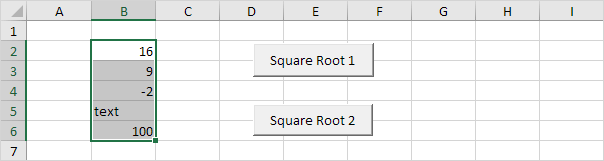### Square Root 1

Add the following code lines to the 'Square Root 1' command button.

1. First, we declare two Range objects. We call the Range objects rng and cell.

Dim rng As Range, cell As Range

2. We initialize the Range object rng with the selected range.

Set rng = Selection

3. We want to calculate the square root of each cell in a randomly selected range (this range can be of any size). In Excel VBA, you can use the For Each Next loop for this. Add the following code lines:

For Each cell In rng

Next cell

Note: rng and cell are randomly chosen here, you can use any names. Remember to refer to these names in the rest of your code.

4. Add the following code line to the loop.

On Error Resume Next

5. Next, we calculate the square root of a value. In Excel VBA, we can use the Sqr function for this. Add the following code line to the loop.

cell.Value = Sqr(cell.Value)

6. Exit the Visual Basic Editor and test the program.

Result: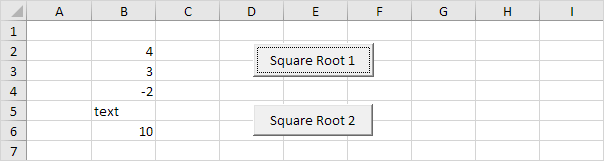Conclusion: Excel VBA has ignored cells containing invalid values such as negative numbers and text. Without using the 'On Error Resume Next' statement you would get two errors. Be careful to only use the 'On Error Resume Next' statement when you are sure ignoring errors is OK.

### Square Root 2

Add the following code lines to the 'Square Root 2' command button.

1. The same program as Square Root 1 but replace 'On Error Resume Next' with:

On Error GoTo InvalidValue:

Note: InvalidValue is randomly chosen here, you can use any name. Remember to refer to this name in the rest of your code.

2. Outside the For Each Next loop, first add the following code line:

Exit Sub

Without this line, the rest of the code (error code) will be executed, even if there is no error!

3. Excel VBA continues execution at the line starting with 'InvalidValue:' upon hitting an error (don't forget the colon). Add the following code line:

InvalidValue:

4. We keep our error code simple for now. We display a MsgBox with some text and the address of the cell where the error occurred.

MsgBox "can't calculate square root at cell " & cell.Address

5. Add the following line to instruct Excel VBA to resume execution after executing the error code.

Resume Next

6. Exit the Visual Basic Editor and test the program.

Result: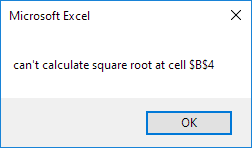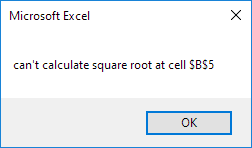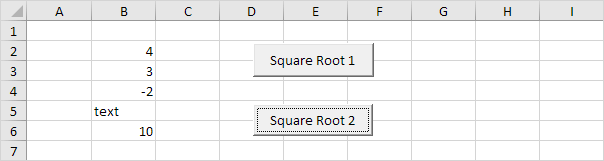Go to Next Chapter: String Manipulation